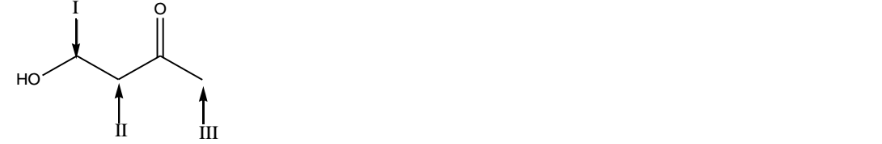# Problem: Which of the following is a correct prediction of the chemical shifts for the signals in the 1H NMR spectrum for the following compound? a) I=3.9 ppm, II=2.2 ppm, III=2.4 ppm b) I=3.7 ppm, II=2.7 ppm, III=1.9 ppm c) I=3.7 ppm, II=2.2 ppm, III=1.9 ppm d) I=3.9 ppm, II=2.7 ppm, III=1.9 ppm e) none of these

93% (1 ratings)
###### Problem Details

Which of the following is a correct prediction of the chemical shifts for the signals in the 1H NMR spectrum for the following compound?

a) I=3.9 ppm, II=2.2 ppm, III=2.4 ppm

b) I=3.7 ppm, II=2.7 ppm, III=1.9 ppm

c) I=3.7 ppm, II=2.2 ppm, III=1.9 ppm

d) I=3.9 ppm, II=2.7 ppm, III=1.9 ppm

e) none of theseWhat scientific concept do you need to know in order to solve this problem?

Our tutors have indicated that to solve this problem you will need to apply the H NMR Table concept. You can view video lessons to learn H NMR Table. Or if you need more H NMR Table practice, you can also practice H NMR Table practice problems.

What is the difficulty of this problem?

Our tutors rated the difficulty ofWhich of the following is a correct prediction of the chemic...as medium difficulty.

How long does this problem take to solve?

Our expert Organic tutor, Chris took 3 minutes and 3 seconds to solve this problem. You can follow their steps in the video explanation above.

What professor is this problem relevant for?

Based on our data, we think this problem is relevant for Professor Ganley's class at MIZZOU.